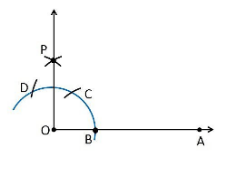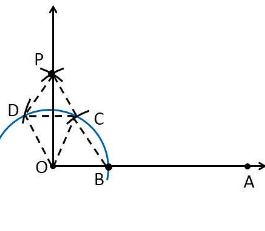# Construct an angle of 90° at the initial point of a given ray and justify the construction.Procedure

To construct an angle of 90°, the given steps can be followed

1. Draw a ray OA

2. Considering O as the centre with any radius, we draw an arc DCB is that cuts OA at B.

3. Considering B as a centre with the same radius, mark a point C on the arc DCB.

4. Considering C as a centre and the same radius, mark a point D on the arc DCB.

5. Let us take C and D as the centre, draw two arcs that intersect each other with the same radius at P.

6. Now join the ray OP making an angle of 90° with OP.

Justification

To prove ∠POA = 90°

To prove this, draw a dotted line from the point O to C and O to D and the angles formed are:From the construction, we note that

OB = BC = OC

Hence, OBC is an equilateral triangle

So that, ∠BOC = 60°.

Likewise

OD = DC = OC

Hence, DOC is an equilateral triangle

So that, ∠DOC = 60°.

From SSS triangle congruence rule

△OBC ≅ OCD

So, ∠BOC = ∠DOC [By C.P.C.T]

Hence, ∠COP = ½ ∠DOC = ½ (60°).

∠COP = 30°

To determine ∠POA = 90°:

∠POA = ∠BOC+∠COP

∠POA = 60°+30°

∠POA = 90°

Hence, Proved